$$\require{cancel}$$

# 4.3 Angular Momentum In Three Dimensions

•• Contributed by Benjamin Crowell
• Professor (Physics) at Fullerton College

Conservation of angular momentum produces some surprising phenomena when extended to three dimensions. Try the following experiment, for example. Take off your shoe, and toss it in to the air, making it spin along its long (toe-to-heel) axis. You should observe a nice steady pattern of rotation. The same happens when you spin the shoe about its shortest (top-to-bottom) axis. But something unexpected happens when you spin it about its third (left-to-right) axis, which is intermediate in length between the other two. Instead of a steady pattern of rotation, you will observe something more complicated, with the shoe changing its orientation with respect to the rotation axis.

# 4.3.1 Rigid-body kinematics in three dimensions

How do we generalize rigid-body kinematics to three dimensions? When we wanted to generalize the kinematics of a moving particle to three dimensions, we made the numbers $$r$$, $$v$$, and $$a$$ into vectors r, v, and a. This worked because these quantities all obeyed the same laws of vector addition. For instance, one of the laws of vector addition is that, just like addition of numbers, vector addition gives the same result regardless of the order of the two quantities being added. Thus you can step sideways 1 m to the right and then step forward 1 m, and the end result is the same as if you stepped forward first and then to the side. In order words, it didn't matter whether you took $$\Delta\mathbf{r}_1+\Delta\mathbf{r}_2$$ or $$\Delta\mathbf{r}_2+\Delta\mathbf{r}_1$$. In math this is called the commutative property of addition.a / Performing the rotations in one order gives one result, 3, while reversing the order gives a different result, 5.

Angular motion, unfortunately doesn't have this property, as shown in figure a. Doing a rotation about the $$x$$ axis and then about $$y$$ gives one result, while doing them in the opposite order gives a different result. These operations don't “commute,” i.e., it makes a difference what order you do them in.

This means that there is in general no possible way to construct a $$\Delta\boldsymbol{\theta}$$ vector. However, if you try doing the operations shown in figure a using small rotation, say about 10 degrees instead of 90, you'll find that the result is nearly the same regardless of what order you use; small rotations are very nearly commutative. Not only that, but the result of the two 10-degree rotations is about the same as a single, somewhat larger, rotation about an axis that lies symmetrically at between the $$x$$ and $$y$$ axes at 45 degree angles to each one. This is exactly what we would expect if the two small rotations did act like vectors whose directions were along the axis of rotation. We therefore define a $$d\boldsymbol{\theta}$$ vector whose magnitude is the amount of rotation in units of radians, and whose direction is along the axis of rotation. Actually this definition is ambiguous, because there it could point in either direction along the axis.b / The right-hand rule for associating a vector with a direction of rotation.

We therefore use a right-hand rule as shown in figure b to define the direction of the $$d\boldsymbol{\theta}$$ vector, and the $$\boldsymbol{\omega}$$ vector, $$\boldsymbol{\omega}=d\boldsymbol{\theta}/dt$$, based on it. Aliens on planet Tammyfaye may decide to define it using their left hands rather than their right, but as long as they keep their scientific literature separate from ours, there is no problem. When entering a physics exam, always be sure to write a large warning note on your left hand in magic marker so that you won't be tempted to use it for the right-hand rule while keeping your pen in your right.

self-check:

Use the right-hand rule to determine the directions of the $$\boldsymbol{\omega}$$ vectors in each rotation shown in figures a/1 through a/5.

Because the vector relationships among $$d\boldsymbol{\theta}$$, $$\boldsymbol{\omega}$$, and $$\boldsymbol{\alpha}$$ are strictly analogous to the ones involving $$d\mathbf{r}$$, $$\mathbf{v}$$, and $$\mathbf{a}$$ (with the proviso that we avoid describing large rotations using $$\Delta\boldsymbol{\theta}$$ vectors), any operation in $$\mathbf{r}$$-$$\mathbf{v}$$-$$\mathbf{a}$$ vector kinematics has an exact analog in $$\boldsymbol{\theta}$$-$$\boldsymbol{\omega}$$-$$\boldsymbol{\alpha}$$ kinematics.

Example 23: Result of successive 10-degree rotations

$$\triangleright$$ What is the result of two successive (positive) 10-degree rotations about the $$x$$ and $$y$$ axes? That is, what single rotation about a single axis would be equivalent to executing these in succession?

$$\triangleright$$ The result is only going to be approximate, since 10 degrees is not an infinitesimally small angle, and we are not told in what order the rotations occur. To some approximation, however, we can add the $$\Delta\boldsymbol{\theta}$$ vectors in exactly the same way we would add $$\Delta \mathbf{r}$$ vectors, so we have

\begin{align*} \Delta\boldsymbol{\theta} &\approx \Delta\boldsymbol{\theta}_1 + \Delta\boldsymbol{\theta}_2 \\ &\approx \text{(10 degrees)}\hat{\mathbf{x}} + \text{(10 degrees)}\hat{\mathbf{y}} . \end{align*}

This is a vector with a magnitude of $$\sqrt{\text{(10 deg)}^2+\text{(10 deg)}^2}=\text{14\ deg}$$, and it points along an axis midway between the $$x$$ and $$y$$ axes.

# 4.3.2 Angular momentum in three dimensions

## The vector cross product

In order to expand our system of three-dimensional kinematics to include dynamics, we will have to generalize equations like $$v_t=\omega r$$, $$\tau=rF \sin\theta_{rF}$$, and $$L=rp \sin\theta_{rp}$$, each of which involves three quantities that we have either already defined as vectors or that we want to redefine as vectors. Although the first one appears to differ from the others in its form, it could just as well be rewritten as $$v_t=\omega r \sin\theta_{\omega r}$$, since $$\theta_{\omega r}=90°$$, and $$\sin\theta_{\omega r}=1$$.

It thus appears that we have discovered something general about the physically useful way to relate three vectors in a multiplicative way: the magnitude of the result always seems to be proportional to the product of the magnitudes of the two vectors being “multiplied,” and also to the sine of the angle between them.

Is this pattern just an accident? Actually the sine factor has a very important physical property: it goes to zero when the two vectors are parallel. This is a Good Thing. The generalization of angular momentum into a three-dimensional vector, for example, is presumably going to describe not just the clockwise or counterclockwise nature of the motion but also from which direction we would have to view the motion so that it was clockwise or counterclockwise. (A clock's hands go counterclockwise as seen from behind the clock, and don't rotate at all as seen from above or to the side.) Now suppose a particle is moving directly away from the origin, so that its r and p vectors are parallel. It is not going around the origin from any point of view, so its angular momentum vector had better be zero.

Thinking in a slightly more abstract way, we would expect the angular momentum vector to point perpendicular to the plane of motion, just as the angular velocity vector points perpendicular to the plane of motion. The plane of motion is the plane containing both r and p, if we place the two vectors tail-to-tail. But if r and p are parallel and are placed tail-to-tail, then there are infinitely many planes containing them both. To pick one of these planes in preference to the others would violate the symmetry of space, since they should all be equally good. Thus the zero-if-parallel property is a necessary consequence of the underlying symmetry of the laws of physics.

The following definition of a kind of vector multiplication is consistent with everything we've seen so far, and on p. 912 we'll prove that the definition is unique, i.e., if we believe in the symmetry of space, it is essentially the only way of defining the multiplication of two vectors to produce a third vector:

\mythmhdr{Definition of the vector cross product}
The cross product $$\mathbf{A}\times\mathbf{B}$$ of two vectors $$\mathbf{A}$$ and $$\mathbf{B}$$ is defined as follows:

1. Its magnitude is defined by $$|\mathbf{A}\times\mathbf{B}| = |\mathbf{A}| |\mathbf{B}| \sin\theta_{AB}$$, where $$\theta_{AB}$$ is the angle between $$\mathbf{A}$$ and $$\mathbf{B}$$ when they are placed tail-to-tail.
2. Its direction is along the line perpendicular to both $$\mathbf{A}$$ and $$\mathbf{B}$$. Of the two such directions, it is the one that obeys the right-hand rule shown in figure c.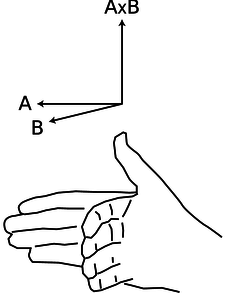Figure c: The right-hand rule for the direction of the vector cross product.

The name “cross product” refers to the symbol, and distinguishes it from the dot product, which acts on two vectors but produces a scalar.

Although the vector cross-product has nearly all the properties of numerical multiplication, e.g., $$\mathbf{A}\times(\mathbf{B}+\mathbf{C}) = \mathbf{A}\times\mathbf{B}+\mathbf{A}\times\mathbf{C}$$, it lacks the usual property of commutativity. Try applying the right-hand rule to find the direction of the vector cross product $$\mathbf{B}\times\mathbf{A}$$ using the two vectors shown in the figure. This requires starting with a flattened hand with the four fingers pointing along $$\mathbf{B}$$, and then curling the hand so that the fingers point along $$\mathbf{A}$$. The only possible way to do this is to point your thumb toward the floor, in the opposite direction. Thus for the vector cross product we have

$\begin{equation*} \mathbf{A}\times\mathbf{B} = -\mathbf{B}\times\mathbf{A} , \end{equation*}$

a property known as anticommutativity. The vector cross product is also not associative, i.e., $$\mathbf{A}\times(\mathbf{B}\times\mathbf{C})$$ is usually not the same as $$(\mathbf{A}\times\mathbf{B})\times\mathbf{C}$$.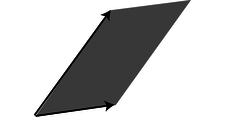d / The magnitude of the cross product is the area of the shaded parallelogram.

A geometric interpretation of the cross product, d, is that if both $$\mathbf{A}$$ and $$\mathbf{B}$$ are vectors with units of distance, then the magnitude of their cross product can be interpreted as the area of the parallelogram they form when placed tail-to-tail.

A useful expression for the components of the vector cross product in terms of the components of the two vectors being multiplied is as follows:

\begin{align*} (\mathbf{A}\times\mathbf{B})_x &= A_yB_z - B_yA_z\\ (\mathbf{A}\times\mathbf{B})_y &= A_zB_x - B_zA_x\\ (\mathbf{A}\times\mathbf{B})_z &= A_xB_y - B_xA_y \end{align*}

I'll prove later that these expressions are equivalent to the previous definition of the cross product. Although they may appear formidable, they have a simple structure: the subscripts on the right are the other two besides the one on the left, and each equation is related to the preceding one by a cyclic change in the subscripts, e. If the subscripts were not treated in some completely symmetric manner like this, then the definition would provide some way to distinguish one axis from another, which would violate the symmetry of space.e / A cyclic change in the $$x$$, $$y$$, and $$z$$ subscripts.

self-check:

Show that the component equations are consistent with the rule $$\mathbf{A}\times\mathbf{B} = -\mathbf{B}\times\mathbf{A}$$.

## Angular momentum in three dimensions

In terms of the vector cross product, we have:

\begin{align*} \mathbf{v} &= \boldsymbol{\omega} \times \mathbf{r}\\ \mathbf{L} &= \mathbf{r} \times \mathbf{p}\\ \boldsymbol{\tau} &= \mathbf{r} \times \mathbf{F} \end{align*}

But wait, how do we know these equations are even correct? For instance, how do we know that the quantity defined by $$\mathbf{r}\times\mathbf{p}$$ is in fact conserved? Well, just as we saw on page 212 that the dot product is unique (i.e., can only be defined in one way while observing rotational invariance), the cross product is also unique, as proved on page 912. If $$\mathbf{r}\times\mathbf{p}$$ was not conserved, then there could not be any generally conserved quantity that would reduce to our old definition of angular momentum in the special case of plane rotation. This doesn't prove conservation of angular momentum --- only experiments can prove that --- but it does prove that if angular momentum is conserved in three dimensions, there is only one possible way to generalize from two dimensions to three.

Example 24: Angular momentum of a spinning topf / The position and momentum vectors of an atom in the spinning top.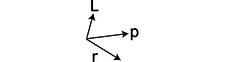g / The right-hand rule for the atom's contribution to the angular momentum.

As an illustration, we consider the angular momentum of a spinning top. Figures f and g show the use of the vector cross product to determine the contribution of a representative atom to the total angular momentum. Since every other atom's angular momentum vector will be in the same direction, this will also be the direction of the total angular momentum of the top. This happens to be rigid-body rotation, and perhaps not surprisingly, the angular momentum vector is along the same direction as the angular velocity vector.

Three important points are illustrated by this example: (1) When we do the full three-dimensional treatment of angular momentum, the “axis” from which we measure the position vectors is just an arbitrarily chosen point. If this had not been rigid-body rotation, we would not even have been able to identify a single line about which every atom circled. (2) Starting from figure f, we had to rearrange the vectors to get them tail-to-tail before applying the right-hand rule. If we had attempted to apply the right-hand rule to figure f, the direction of the result would have been exactly the opposite of the correct answer. (3) The equation $$\mathbf{L}=\mathbf{r}\times\mathbf{p}$$ cannot be applied all at once to an entire system of particles. The total momentum of the top is zero, which would give an erroneous result of zero angular momentum (never mind the fact that r is not well defined for the top as a whole).

Doing the right-hand rule like this requires some practice. I urge you to make models like g out of rolled up pieces of paper and to practice with the model in various orientations until it becomes natural.

Example 25: Precessionh / A top is supported at its tip by a pinhead. (More practical devices to demonstrate this would use a double bearing.)

Figure h shows a counterintuitive example of the concepts we've been discussing. One expects the torque due to gravity to cause the top to flop down. Instead, the top remains spinning in the horizontal plane, but its axis of rotation starts moving in the direction shown by the shaded arrow. This phenomenon is called precession.i / The torque made by gravity is in the horizontal plane.

Figure i shows that the torque due to gravity is out of the page. (Actually we should add up all the torques on all the atoms in the top, but the qualitative result is the same.) Since torque is the rate of change of angular momentum, $$\boldsymbol{\tau}=d\mathbf{L}/dt$$, the $$\Delta\mathbf{L}$$ vector must be in the same direction as the torque (division by a positive scalar doesn't change the direction of the vector).j / The $$\Delta\mathbf{L}$$ vector is in the same direction as the torque, out of the page.

As shown in j, this causes the angular momentum vector to twist in space without changing its magnitude.

For similar reasons, the Earth's axis precesses once every 26,000 years (although not through a great circle, since the angle between the axis and the force isn't 90 degrees as in figure h). This precession is due to a torque exerted by the moon. If the Earth was a perfect sphere, there could be no precession effect due to symmetry. However, the Earth's own rotation causes it to be slightly flattened (oblate) relative to a perfect sphere, giving it “love handles” on which the moon's gravity can act. The moon's gravity on the nearer side of the equatorial bulge is stronger, so the torques do not cancel out perfectly. Presently the earth's axis very nearly lines up with the star Polaris, but in 12,000 years, the pole star will be Vega instead.

Example 26: The frisbee

The flow of the air over a flying frisbee generates lift, and the lift at the front and back of the frisbee isn't necessarily balanced. If you throw a frisbee without rotating it, as if you were shooting a basketball with two hands, you'll find that it pitches, i.e., its nose goes either up or down. When I do this with my frisbee, it goes nose down, which apparently means that the lift at the back of the disc is greater than the lift at the front. The two torques are unbalanced, resulting in a total torque that points to the left.

The way you actually throw a frisbee is with one hand, putting a lot of spin on it. If you throw backhand, which is how most people first learn to do it, the angular momentum vector points down (assuming you're right-handed). On my frisbee, the aerodynamic torque to the left would therefore tend to make the angular momentum vector precess in the clockwise direction as seen by the thrower. This would cause the disc to roll to the right, and therefore follow a curved trajectory. Some specialized discs, used in the sport of disc golf, are actually designed intentionally to show this behavior; they're known as “understable” discs. However, the typical frisbee that most people play with is designed to be stable: as the disc rolls to one side, the airflow around it is altered in way that tends to bring the disc back into level flight. Such a disc will therefore tend to fly in a straight line, provided that it is thrown with enough angular momentum.

Example 27: Finding a cross product by components

$$\triangleright$$ What is the torque produced by a force given by $$\hat{\mathbf{x}}+2\hat{\mathbf{y}}+3\hat{\mathbf{z}}$$ (in units of Newtons) acting on a point whose radius vector is $$4\hat{\mathbf{x}}+2\hat{\mathbf{y}}$$ (in meters)?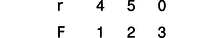k / Example 27.

$$\triangleright$$ It's helpful to make a table of the components as shown in the figure. The results are \begin{alignat*}{2} \tau_x &= r_{y} F_{z} - F_y r_{z} = 15\ N\!\cdot\! m \\
\tau_y &= r_{z} F_x - F_{z} r_{x} = - 12\ N\!\cdot\! m \\
\tau_z &= r_x F_{y} - F_{x} r_y = 3\ N\!\cdot\! m \end{alignat*}

Example 28: Torque and angular momentum

In this example, we prove explicitly the consistency of the equations involving torque and angular momentum that we proved above based purely on symmetry. Starting from the definition of torque, we have

\begin{align*} \boldsymbol{\tau} &= \frac{d\mathbf{L}}{dt} \\ &= \frac{d}{dt}\sum_{i} \mathbf{r}_i\times\mathbf{p}_i \\ &= \sum_{i} \frac{d}{dt}(\mathbf{r}_i\times\mathbf{p}_{i}) . \end{align*}

The derivative of a cross product can be evaluated in the same way as the derivative of an ordinary scalar product:

$\begin{equation*} \boldsymbol{\tau} = \sum_i\left[ \left(\frac{d\mathbf{r}_{i}}{dt}\times\mathbf{p}_i\right) +\left(\mathbf{r}_i\times\frac{d\mathbf{p}_{i}}{dt}\right) \right] \end{equation*}$

The first term is zero for each particle, since the velocity vector is parallel to the momentum vector. The derivative appearing in the second term is the force acting on the particle, so

$\begin{equation*} \boldsymbol{\tau} = \sum_i \mathbf{r}_{i}\times\mathbf{F}_i , \end{equation*}$

which is the relationship we set out to prove.

# 4.3.3 Rigid-body dynamics in three dimensions

The student who is not madly in love with mathematics may wish to skip the rest of this section after absorbing the statement that, for a typical, asymmetric object, the angular momentum vector and the angular velocity vector need not be parallel. That is, only for a body that possesses symmetry about the rotation axis is it true that $$\mathbf{L}=I\boldsymbol{\omega}$$ (the rotational equivalent of $$\mathbf{p}=m\mathbf{v}$$) for some scalar $$I$$.

Let's evaluate the angular momentum of a rigidly rotating system of particles:

\begin{align*} \mathbf{L} &= \sum_i \mathbf{r}_i \times \mathbf{p}_i \\ &= \sum_i m_i \mathbf{r}_i \times \mathbf{v}_i \\ &= \sum_i m_i \mathbf{r}_i \times (\boldsymbol{\omega} \times \mathbf{r}_i) \end{align*}

An important mathematical skill is to know when to give up and back off. This is a complicated expression, and there is no reason to expect it to simplify and, for example, take the form of a scalar multiplied by $$\omega$$. Instead we examine its general characteristics. If we expanded it using the equation that gives the components of a vector cross product, every term would have one of the $$\omega$$ components raised to the first power, multiplied by a bunch of other stuff. The most general possible form for the result is

\begin{align*} L_x &= I_{xx}\omega_x + I_{xy}\omega_y + I_{xz}\omega_z\\ L_y &= I_{yx}\omega_x + I_{yy}\omega_y + I_{yz}\omega_z\\ L_z &= I_{zx}\omega_x + I_{zy}\omega_y + I_{zz}\omega_z , \end{align*}

which you may recognize as a case of matrix multiplication. The moment of inertia is not a scalar, and not a three-component vector. It is a matrix specified by nine numbers, called its matrix elements.

The elements of the moment of inertia matrix will depend on our choice of a coordinate system. In general, there will be some special coordinate system, in which the matrix has a simple diagonal form: \begin{alignat*}{2} L_x &= I_{xx}\omega_x & &
L_y &= & I_{yy}\omega_y &
L_z &= & & I_{zz}\omega_z . \end{alignat*}

The three special axes that cause this simplification are called the principal axes of the object, and the corresponding coordinate system is the principal axis system. For symmetric shapes such as a rectangular box or an ellipsoid, the principal axes lie along the intersections of the three symmetry planes, but even an asymmetric body has principal axes.

We can also generalize the plane-rotation equation $$K=(1/2)I\omega^2$$ to three dimensions as follows:

\begin{align*} K &= \sum_i \frac{1}{2} m_i v_i^2 \\ & = \frac{1}{2} \sum_i m_i (\boldsymbol{\omega} \times \mathbf{r}_i) \cdot (\boldsymbol{\omega} \times \mathbf{r}_i) \end{align*}

We want an equation involving the moment of inertia, and this has some evident similarities to the sum we originally wrote down for the moment of inertia. To massage it into the right shape, we need the vector identity $$(\mathbf{A}\times\mathbf{B})\cdot\mathbf{C}= (\mathbf{B}\times\mathbf{C}) \cdot \mathbf{A}$$, which we state without proof. We then write

\begin{align*} K & = \frac{1}{2} \sum_i m_i \left[ \mathbf{r}_i \times (\boldsymbol{\omega} \times \mathbf{r}_i) \right] \cdot \boldsymbol{\omega} \\ &= \frac{1}{2} \boldsymbol{\omega} \cdot \sum_i m_i\mathbf{r}_i \times (\boldsymbol{\omega} \times \mathbf{r}_i) \\ &= \frac{1}{2} \mathbf{L} \cdot \boldsymbol{\omega} \end{align*}

As a reward for all this hard work, let's analyze the problem of the spinning shoe that I posed at the beginning of the chapter. The three rotation axes referred to there are approximately the principal axes of the shoe. While the shoe is in the air, no external torques are acting on it, so its angular momentum vector must be constant in magnitude and direction. Its kinetic energy is also constant. That's in the room's frame of reference, however. The principal axis frame is attached to the shoe, and tumbles madly along with it. In the principal axis frame, the kinetic energy and the magnitude of the angular momentum stay constant, but the actual direction of the angular momentum need not stay fixed (as you saw in the case of rotation that was initially about the intermediate-length axis). Constant $$|\mathbf{L}|$$ gives

$\begin{equation*} L_x^2 + L_y^2+ L_z^2 = \text{constant} . \end{equation*}$

In the principal axis frame, it's easy to solve for the components of $$\boldsymbol{\omega}$$ in terms of the components of $$\mathbf{L}$$, so we eliminate $$\omega$$ from the expression $$2K=\mathbf{L}\cdot\boldsymbol{\omega}$$, giving

$\begin{equation*} \frac{1}{I_{xx}}L_x^2 + \frac{1}{I_{yy}}L_y^2 + \frac{1}{I_{zz}}L_z^2 = \text{constant #2} . \end{equation*}$

The first equation is the equation of a sphere in the three dimensional space occupied by the angular momentum vector, while the second one is the equation of an ellipsoid.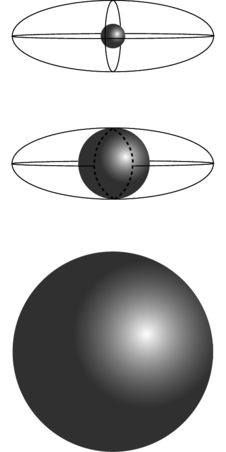l / Visualizing surfaces of constant energy and angular momentum in $$L_x$$-$$L_y$$-$$L_z$$ space.

The top figure corresponds to the case of rotation about the shortest axis, which has the greatest moment of inertia element. The intersection of the two surfaces consists only of the two points at the front and back of the sphere. The angular momentum is confined to one of these points, and can't change its direction, i.e., its orientation with respect to the principal axis system, which is another way of saying that the shoe can't change its orientation with respect to the angular momentum vector. In the bottom figure, the shoe is rotating about the longest axis. Now the angular momentum vector is trapped at one of the two points on the right or left. In the case of rotation about the axis with the intermediate moment of inertia element, however, the intersection of the sphere and the ellipsoid is not just a pair of isolated points but the curve shown with the dashed line. The relative orientation of the shoe and the angular momentum vector can and will change.

One application of the moment of inertia tensor is to video games that simulate car racing or flying airplanes.

One more exotic example has to do with nuclear physics. Although you have probably visualized atomic nuclei as nothing more than featureless points, or perhaps tiny spheres, they are often ellipsoids with one long axis and two shorter, equal ones. Although a spinning nucleus normally gets rid of its angular momentum via gamma ray emission within a period of time on the order of picoseconds, it may happen that a deformed nucleus gets into a state in which has a large angular momentum is along its long axis, which is a very stable mode of rotation. Such states can live for seconds or even years! (There is more to the story --- this is the topic on which I wrote my Ph.D. thesis --- but the basic insight applies even though the full treatment requires fancy quantum mechanics.)

Our analysis has so far assumed that the kinetic energy of rotation energy can't be converted into other forms of energy such as heat, sound, or vibration. When this assumption fails, then rotation about the axis of least moment of inertia becomes unstable, and will eventually convert itself into rotation about the axis whose moment of inertia is greatest. This happened to the U.S.'s first artificial satellite, Explorer I, launched in 1958. Note the long, floppy antennas, which tended to dissipate kinetic energy into vibration. It had been designed to spin about its minimimum-moment-of-inertia axis, but almost immediately, as soon as it was in space, it began spinning end over end. It was nevertheless able to carry out its science mission, which didn't depend on being able to maintain a stable orientation, and it discovered the Van Allen radiation belts.m / The Explorer I satellite.

# Contributors

Benjamin Crowell (Fullerton College). Conceptual Physics is copyrighted with a CC-BY-SA license.